# BITSAT Mathematics Sequences and Series MCQs

BITSAT Mathematics Sequences and Series MCQs with answers available in Pdf for free download. The MCQ Questions for BITSAT Mathematics with answers have been prepared as per the latest BITSAT Mathematics syllabus, books and examination pattern. Multiple Choice Questions form important part of competitive exams and BITSAT exam and if practiced properly can help you to get higher rank. Refer to more topic wise BITSAT Mathematics Questions and also download more latest study material for all subjects and do free BITSAT Mathematics Mock Test

## MCQ for BITSAT Mathematics Sequences and Series

BITSAT Mathematics students should refer to the following multiple-choice questions with answers for Sequences and Series in BITSAT. These MCQ questions with answers for BITSAT Mathematics will come in exams and help you to score good marks

### Sequences and Series MCQ Questions with Answers

#### Question: If A, B, C are the angles of a triangle and eiA,eiB,eiC are in A.P. Then the triangle must be

• a) Right angled
• b) Isosceles
• c) Equilateral
• d) None of these

#### where p, q, t and s are constants, then the value of s is equal to

• a)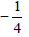• b)• c)• d)• a) 960 m
• b) 1000 m
• c) 1080 m
• d) Infinite

• a) 1
• b) 2
• c) 3/2
• d) 5/2

#### Question: The fourth term of an A.P. is three times of the first term and the seventh term exceeds the twice of the third term by one, then the common difference of the progression is

• a) 2
• b) 3
• c)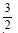• d) -1

#### Question: The sum to n terms of the series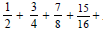__________ is

• a) n – 1 – 2– n
• b) 1
• c) n – 1 + 2– n
• d) 1 + 2– n

Answer: n – 1 + 2– n

#### Question: If log a, log b, and log c are in A.P. and also log a– log 2b, log 2b – log 3c, log 3c – log a are in A.P., then

• a) a, b, c, are in H.P
• b) a, 2b, 3c are in A.P.
• c) a, b, c are the sides of a triangle
• d) None of these

Answer: a, b, c are the sides of a triangle

#### Question:upto n terms is

• a)• b)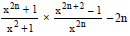• c)• d) None of these• a) -1
• b) 1
• c) -i
• d) i

#### ____ upto n terms is

• a)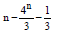• b)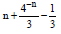• c)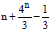• d)#### Question: If binomial coefficients of three consecutive terms of (1 + x)n are in HP, then the maximum value of n is

• a) 1
• b) 2
• c) 0
• d) None of these

#### Question: If the (2p)th term of a H.P. is q and the (2q)th term is p, then the 2(p + q)th term is-

• a)• b)• c)• d)#### Question: Ifare A. P., then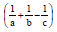is equal to

• a)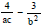• b)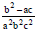• c)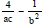• d) None of these#### Question: The product of n positive numbers is unity, then their sum is :

• a) A positive integer
• b) Divisible by n
• c)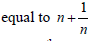• d) Never less than n

#### Question: Let Tr be the rth term of an A.P. for r = 1, 2, 3, .... If for some positive integers m, n we have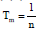andthen Tmn equals

• a)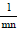• b)• c) 1
• d) 0

#### Question: If x is positive then the sum to infinity of the series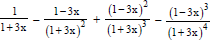........... ∞ is

• a) 1/2
• b)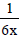• c)• d)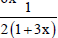• a) 2
• b) 0
• c) 4
• d) 8

• a) 10
• b) 12
• c) 11
• d) 13

• a) 14
• b) 24
• c) 10
• d) 20

• a) 12
• b) 13
• c) 14
• d) 15

• a) 1
• b) 2
• c) 3
• d) 4

#### Question: Find the geometric mean of numbers 7, 72, 73,.......,7n

• a)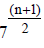• b)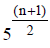• c)• d)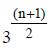• a) 178090
• b) 168090
• c) 188090
• d) 190090

#### Question: The sum of infinite terms of the geometric progression............is

• a) √2( √2 +1)2
• b) ( √2 +1)2
• c) 5 √2
• d) 3 √2 + √5

#### Question: If the non-zero numbers x, y, z are in A. P. and tan –1x, tan –1y, tan –1 z are in A. P. then

• a) x = y = z
• b) y = zx
• c) x = yz
• d) z = xy

Answer: x = y = z

• a) -5 √2
• b) 5 √2
• c) 10 √2
• d) -10 √2

• a) 6
• b) 12
• c) 10
• d) 8

• a) 10
• b) 12
• c) 11
• d) 13

• a) 14
• b) 24
• c) 10
• d) 20

• a) 12
• b) 13
• c) 14
• d) 15